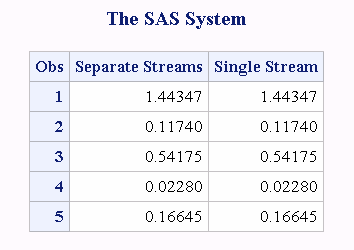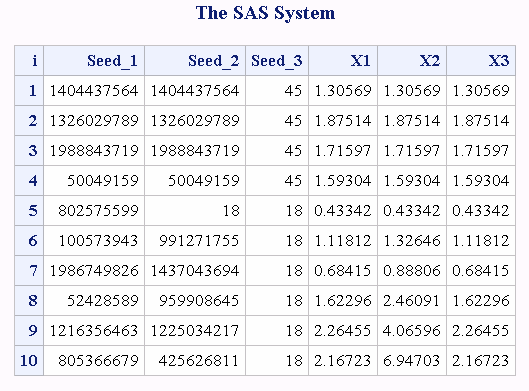# CALL RANGAM Routine

Returns a random variate from a gamma distribution.

 Category: Random Number

## Syntax

CALL RANGAM(seed,a,x);

### Required Arguments

#### seed

is the seed value. A new value for seed is returned each time CALL RANGAM is executed.

 Range seed < 231 - 1 Note If seed ≤ 0, the time of day is used to initialize the seed stream. See Seed Values and Comparison of Seed Values in Random-Number Functions and CALL Routines for more information about seed values

#### a

is a numeric shape parameter.

 Range a > 0

#### x

is a numeric variable. A new value for the random variate x is returned each time CALL RANGAM is executed.

## Details

The CALL RANGAM routine updates seed and returns a variate x that is generated from a gamma distribution with parameter a.
By adjusting the seeds, you can force streams of variates to agree or disagree for some or all of the observations in the same, or in subsequent, DATA steps.
For a>1, an acceptance-rejection method by Cheng is used (Cheng, 1977). For a ≤ 1, an acceptance-rejection method by Fishman is used (Fishman, 1978). For more information see .
For a discussion and example of an effective use of the random number CALL routines, see Starting. Stopping, and Restarting a Stream.

## Comparisons

The CALL RANGAM routine gives greater control of the seed and random number streams than does the RANGAM function.

## Example

This example uses the CALL RANGAM routine:
```data u1(keep=x);
seed = 104;
do i = 1 to 5;
call rangam(seed, 1, x);
output;
end;
call symputx('seed', seed);
run;

data u2(keep=x);
seed = &seed
do i = 1 to 5;
call rangam(seed, 1, x);
output;
end;
run;

data all;
set u1 u2;
z = rangam(104, 1);
run;

proc print label;
label x = 'Separate Streams' z = 'Single Stream';
run;
```
Output from the CALL RANGAM RoutineThis is another example that uses the CALL RANGAM routine:
```data case;
retain Seed_1 Seed_2 Seed_3 45;
a=2;
do i=1 to 10;
call rangam(Seed_1,a,X1);
call rangam(Seed_2,a,X2);
X3=rangam(Seed_3,a);
if i=5 then
do;
Seed_2=18;
Seed_3=18;
end;
output;
end;
run;

proc print;
id i;
var Seed_1-Seed_3 X1-X3;
run;
```
Output from the CALL RANGAM RoutineChanging Seed_2 for the CALL RANGAM statement, when I=5, forces the stream of the variates for X2 to deviate from the stream of the variates for X1. Changing Seed_3 on the RANGAM function, however, has no effect.

Functions: